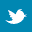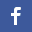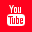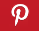Completely Solved C, C++ Programs Assignment.Quick Search Database Videos Tutorials Ebooks Forums FAQ Aboutus Household Industrial Manufacturing Service Shopping Transportation### C program to check the number is prime or not.

 Filed Under: C Assignments

Problem statement:
A ‘C’ program to check the number is prime or not.

Theory:
A prime number (or a prime) is a natural number that has exactly two distinct natural number divisors: 1 and itself. The smallest twenty-five prime numbers (all the prime numbers under 100) are:2, 3, 5, 7, 11, 13, 17, 19, 23, 29, 31, 37, 41, 43, 47, 53, 59, 61, 67, 71, 73, 79, 83, 89, 97. Verifying the primarily of a given number n can be done by division. The simplest trial division method tests whether n is a multiple of an integer m between 2 and . If n is a multiple of any of these integers then it is a composite number, and so not prime; if it is not a multiple of any of these integers then it is prime. it is only suitable for relatively small values of n.

Recursion:
Most high-level computer programming languages support recursion by allowing a function to call itself within the program text. Some functional programming languages do not define any looping constructs but rely solely on recursion to repeatedly call code. Computability theory has proven that these recursive-only languages are mathematically equivalent to the imperative languages, meaning they can solve the same kinds of problems even without the typical control structures like “while” and “for”.

Algorithm:
int prime(int n,int j)                  /*Function definition for checking the number is prime or not*/
{
if(n==j)
return 1;
if(n>j)
{
if(n%j==0)
return 0;
else
return(prime(n,j+1));
}
return 0;
}

PROGRAM LISTING:
#include<stdio.h>
#include<conio.h>
#include<math.h>
int prime(int,int);                                         /*function prototype*/
void main()
{
int c,n,j=2;
clrscr();
printf("\n enter the number: ");
scanf("%d",&n);
c=prime(n,j);                          /*calling the function to check whether the number is prime or not*/
if(c==1)
printf("\n %d is prime",n);
else
printf("\n %d is not prime",n);
getch();
}
int prime(int n,int j)                          /*Function definition for checking the number is prime or not*/
{
if(n==j)
return 1;
if(n>j)
{ if(n%j==0)
return 0;
else
return
(prime(n,j+1));
}
return 0;
}

Output:
enter the number: 13
13 is prime
enter the number: 15
15 is not prime
enter the number: 23
23 is prime
enter the number: 133
133 is not prime
enter the number: 111
111 is not prime

Discussion:
• Recursion in computer science is a method where the solution to a problem depends on solutions to smaller instances of the same problem. The approach can be applied to many types of problems, and is one of the central ideas of computer science.
• Prime numbers give rise to various generalizations in other mathematical domains, mainly algebra, notably the notion of prime ideals.Back to main directory:Software Practical